Question

# Assuming the production function: Q = 2.5L0.4 K0.6 , a wage rate of \$24 and a rental rate of \$18.

Assuming the production function: Q = 2.5L0.4 K0.6 , a wage rate of \$24 and a rental rate of \$18.

a. What is the least costly combination of L and K needed to produce 1000 units?

b. What is the cost of the bundle found in sub-question a?

c. What is the least costly combination need to produce 2000 units?

d. Please illustrate the relationship of L, K, Q in a carefully labelled diagram. (Please use the information from sub-questions a and c)

e. How would your answer to sub-question a change if the rental rate increased to \$24?

We need at least 10 more requests to produce the answer.

0 / 10 have requested this problem solution

The more requests, the faster the answer.

All students who have requested the answer will be notified once they are available.

#### Earn Coins

Coins can be redeemed for fabulous gifts.

Similar Homework Help Questions
• ### 5. Suppose a firm has the production function Q=K1/2 [4M1/4 The wage rate w=16, rental rate...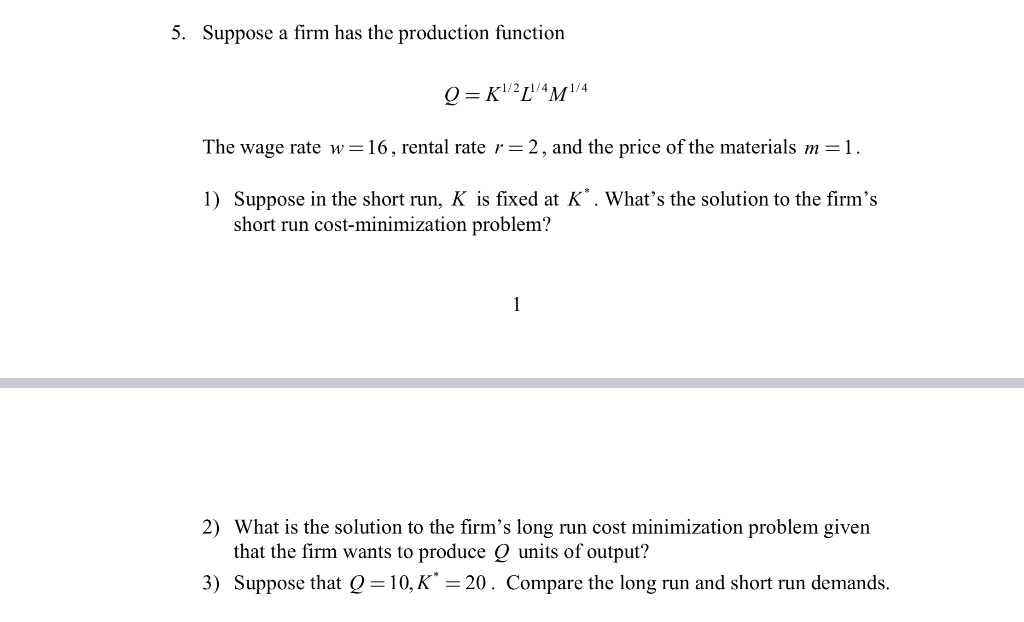5. Suppose a firm has the production function Q=K1/2 [4M1/4 The wage rate w=16, rental rate r=2, and the price of the materials m=1. 1) Suppose in the short run, K is fixed at K*. What's the solution to the firm's short run cost-minimization problem? 2) What is the solution to the firm's long run cost minimization problem given that the firm wants to produce Q units of output? 3) Suppose that Q = 10,K* = 20. Compare the long...

• ### Suppose that a firm had a production function given by q-21 K The rental rate for...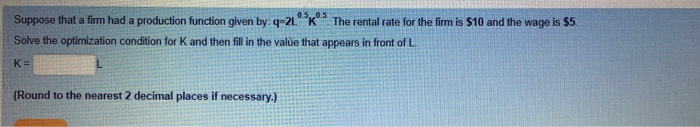Suppose that a firm had a production function given by q-21 K The rental rate for the fim is \$10 and the wage is s5 Solve the optimization condition for K and then fill in the value that appears in front of L K- Round to the nearest 2 decimal places if necessary.) Suppose that a firm had a production function given by q-L025k075. The wage rate (w) is \$10 and the rental rate () is \$10 Calculate the amount...

• ### 6. Suppose the wage is \$8, the rental rate of capital is \$128, and the firm's...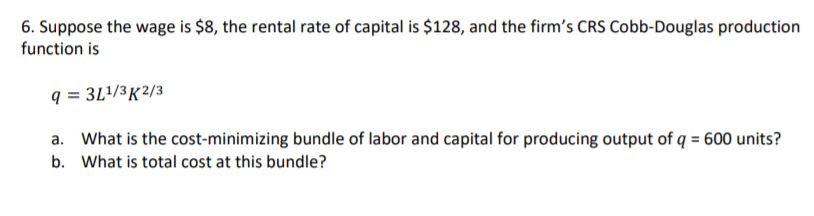6. Suppose the wage is \$8, the rental rate of capital is \$128, and the firm's CRS Cobb-Douglas production function is q = 3L1/3K2/3 a. What is the cost-minimizing bundle of labor and capital for producing output of q = 600 units? b. What is total cost at this bundle?

• ### A firm has a Cobb-Douglas production function of Q = K^(0.25) L^(0.75) (a) Does this production...

A firm has a Cobb-Douglas production function of Q = K^(0.25) L^(0.75) (a) Does this production technology exhibit increasing, constant, or decreasing returns to scale? (b) Suppose that the rental rate of capital is r = 1, the wage rate is w = 1, and the ?rm wants to produce Q = 3. In the long-run, what combination of L and K should they use? (It would be good to practice doing this with the Lagrangian, even if you can...

• ### A firm produces gizmos according to the production function Q =10KL , where Q is the...

A firm produces gizmos according to the production function Q =10KL , where Q is the quantity of gismos produced, K is the quantity of capital rented and L is the quantity of labour hired. The manager has been given a production target: Produce 9,000 gizmos per day. He is informed that the daily rental price of capital is \$400 per unit and the wage rate is \$200 per day. a) Currently, the firm has 10 units of capital. How...

• ### SHOW ALL WORK !!! 6. Suppose the wage is \$8, the rental rate of capital is...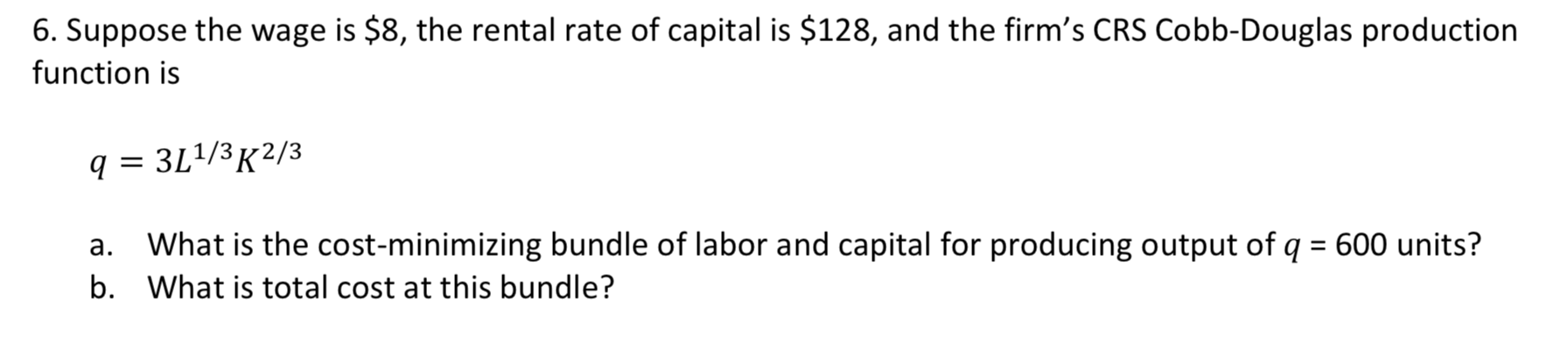SHOW ALL WORK !!! 6. Suppose the wage is \$8, the rental rate of capital is \$128, and the firm's CRS Cobb-Douglas production function is q 3L1/3 K2/3 What is the cost-minimizing bundle of labor and capital for producing output of q = 600 units? а. b. What is total cost at this bundle?

• ### Suppose that a firm had a production function given by: q=L^0.25*K^0.75. The wage rate (w) is...

Suppose that a firm had a production function given by: q=L^0.25*K^0.75. The wage rate (w) is \$10 and the rental rate (r) is \$20. Calculate the amount of labor the firm would hire when it produces 300 units of output in a cost-minimizing way

• ### 5. Suppose a firm has the production function Q = K1/2 L1/4 M1/4 The wage rate...

5. Suppose a firm has the production function Q = K1/2 L1/4 M1/4 The wage rate w = 16 , rental rate r = 2 , and the price of the materials m = 1. 1) Suppose in the short run, K is fixed at K *. What’s the solution to the firm’s short run cost-minimization problem? 2) What is the solution to the firm’s long run cost minimization problem given that the firm wants to produce Q units of...

• ### suppose a firm has a cobb-douglas weekly production function q=f(l,k)=25l^.5k^.5, where l is the number of...

suppose a firm has a cobb-douglas weekly production function q=f(l,k)=25l^.5k^.5, where l is the number of workers and k is units of capital.mrtslk is k/l. the wage rate is \$900 per week, and a unit of capital costs \$400 per week. assuming no fixed cost, what is the firm's total cost of production if it uses least-cost input combination to produce 675 units of output?

• ### 2. Suppose the firm has the one variable production function Q=L?. Assume that the wage rate...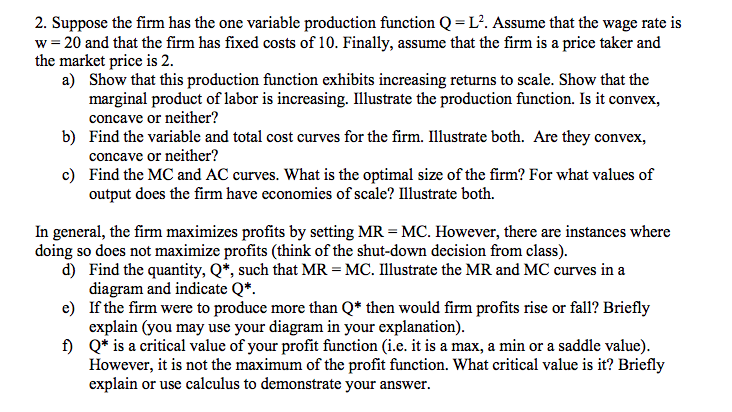2. Suppose the firm has the one variable production function Q=L?. Assume that the wage rate is w= 20 and that the firm has fixed costs of 10. Finally, assume that the firm is a price taker and the market price is 2. a) Show that this production function exhibits increasing returns to scale. Show that the marginal product of labor is increasing. Illustrate the production function. Is it convex, concave or neither? b) Find the variable and total cost...